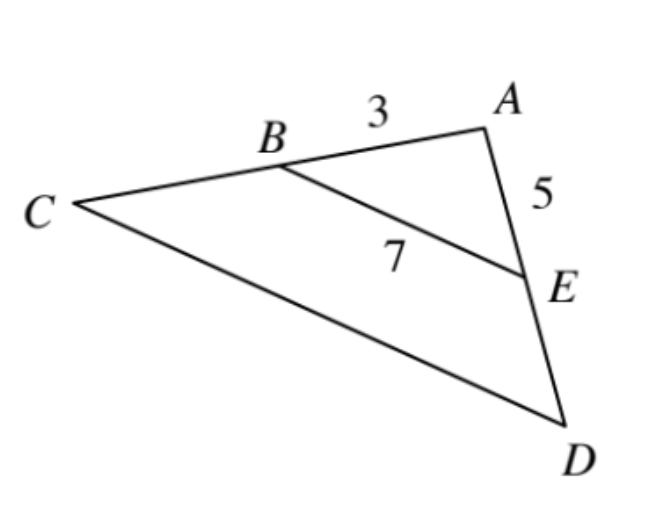### Home > INT2 > Chapter 9 > Lesson 9.3.4 > Problem9-124

9-124.

$\overline { B E }$ is the midsegment of $ΔACD$, shown below.1. Determine the perimeter of $ΔACD$.

We are given values for the lines in this shape. Pay attention to the midsegment of the shape. Can you multiply these variables by a specific number?

$CD = 14$, $BC = 3$, and $ED = 5$
Perimeter $= 14 + 6 + 10 = 30$ units.

2. If the area of $ΔABE$ is $6.5$ units$^2$, what is the area of $ΔACD$?

$(6.5)(4) = 26$ units $^²$